tsne

t-Distributed Stochastic Neighbor Embedding

Description

example

Y = tsne(X) returns a matrix of two-dimensional embeddings of the high-dimensional rows of X.

example

Y = tsne(X,Name,Value) modifies the embeddings using options specified by one or more name-value pair arguments.

example

[Y,loss] = tsne(___), for any input arguments, also returns the Kullback-Leibler divergence between the joint distributions that model the data X and the embedding Y.

Examples

collapse all

The Fisher iris data set has four-dimensional measurements of irises, and corresponding classification into species. Visualize this data by reducing the dimension using tsne.

rng default % for reproducibility
Y = tsne(meas);
gscatter(Y(:,1),Y(:,2),species)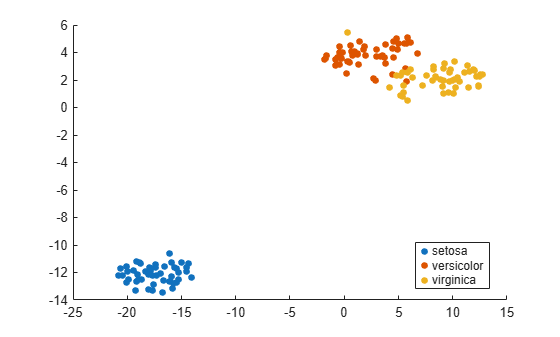Use various distance metrics to try to obtain a better separation between species in the Fisher iris data.

rng('default') % for reproducibility
Y = tsne(meas,'Algorithm','exact','Distance','mahalanobis');
subplot(2,2,1)
gscatter(Y(:,1),Y(:,2),species)
title('Mahalanobis')

rng('default') % for fair comparison
Y = tsne(meas,'Algorithm','exact','Distance','cosine');
subplot(2,2,2)
gscatter(Y(:,1),Y(:,2),species)
title('Cosine')

rng('default') % for fair comparison
Y = tsne(meas,'Algorithm','exact','Distance','chebychev');
subplot(2,2,3)
gscatter(Y(:,1),Y(:,2),species)
title('Chebychev')

rng('default') % for fair comparison
Y = tsne(meas,'Algorithm','exact','Distance','euclidean');
subplot(2,2,4)
gscatter(Y(:,1),Y(:,2),species)
title('Euclidean')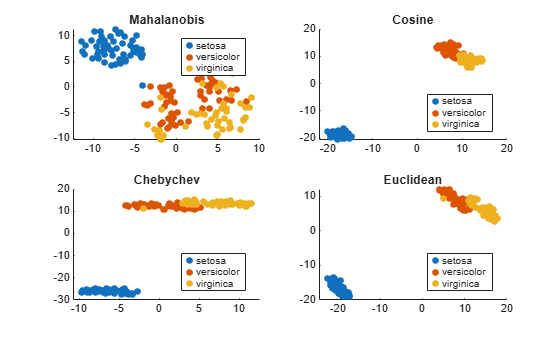In this case, the cosine, Chebychev, and Euclidean distance metrics give reasonably good separation of clusters. But the Mahalanobis distance metric does not give a good separation.

tsne removes input data rows that contain any NaN entries. Therefore, you must remove any such rows from your classification data before plotting.

For example, change a few random entries in the Fisher iris data to NaN.

rng default % for reproducibility
meas(rand(size(meas)) < 0.05) = NaN;

Embed the four-dimensional data into two dimensions using tsne.

Y = tsne(meas,'Algorithm','exact');
Warning: Rows with NaN missing values in X or 'InitialY' values are removed.

Determine how many rows were eliminated from the embedding.

length(species)-length(Y)
ans = 22

Prepare to plot the result by locating the rows of meas that have no NaN values.

goodrows = not(any(isnan(meas),2));

Plot the results using only the rows of species that correspond to rows of meas with no NaN values.

gscatter(Y(:,1),Y(:,2),species(goodrows))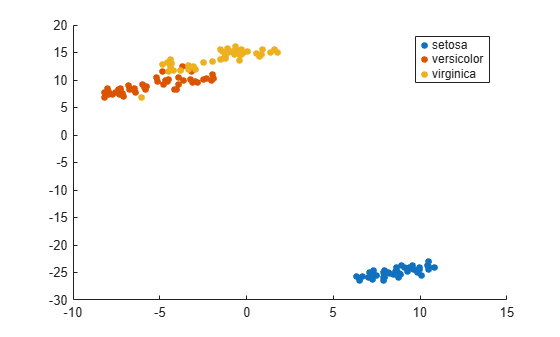Find both 2-D and 3-D embeddings of the Fisher iris data, and compare the loss for each embedding. It is likely that the loss is lower for a 3-D embedding, because this embedding has more freedom to match the original data.

rng default % for reproducibility
[Y,loss] = tsne(meas,'Algorithm','exact');
rng default % for fair comparison
[Y2,loss2] = tsne(meas,'Algorithm','exact','NumDimensions',3);
fprintf('2-D embedding has loss %g, and 3-D embedding has loss %g.\n',loss,loss2)
2-D embedding has loss 0.124191, and 3-D embedding has loss 0.0990884.

As expected, the 3-D embedding has lower loss.

View the embeddings. Use RGB colors [1 0 0], [0 1 0], and [0 0 1].

For the 3-D plot, convert the species to numeric values using the categorical command, then convert the numeric values to RGB colors using the sparse function as follows. If v is a vector of positive integers 1, 2, or 3, corresponding to the species data, then the command

sparse(1:numel(v),v,ones(size(v)))

is a sparse matrix whose rows are the RGB colors of the species.

gscatter(Y(:,1),Y(:,2),species,eye(3))
title('2-D Embedding')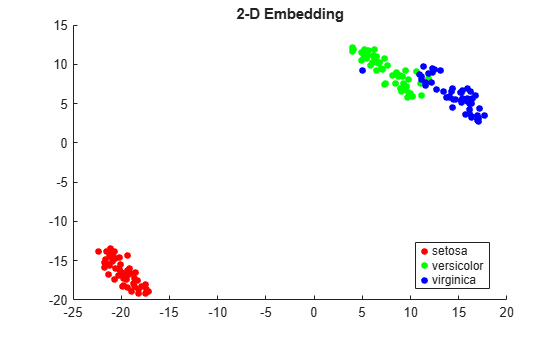figure
v = double(categorical(species));
c = full(sparse(1:numel(v),v,ones(size(v)),numel(v),3));
scatter3(Y2(:,1),Y2(:,2),Y2(:,3),15,c,'filled')
title('3-D Embedding')
view(-50,8)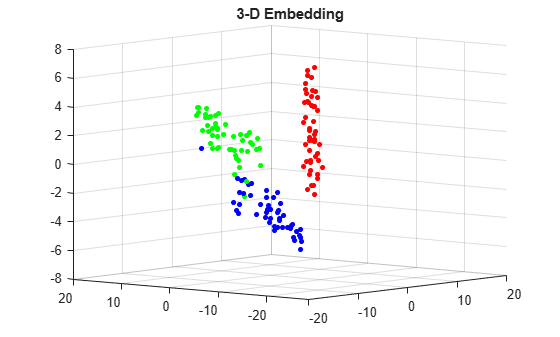Input Arguments

collapse all

Data points, specified as an n-by-m matrix, where each row is one m-dimensional point.

tsne removes rows of X that contain any NaN values before creating an embedding. See Plot Results with NaN Input Data.

Data Types: single | double

Name-Value Arguments

Specify optional comma-separated pairs of Name,Value arguments. Name is the argument name and Value is the corresponding value. Name must appear inside quotes. You can specify several name and value pair arguments in any order as Name1,Value1,...,NameN,ValueN.

Example: Y = tsne(X,'Algorithm','Exact','NumPCAComponents',50)
Algorithm Control

collapse all

tsne algorithm, specified as 'barneshut' or 'exact'. The 'exact' algorithm optimizes the Kullback-Leibler divergence of distributions between the original space and the embedded space. The 'barneshut' algorithm performs an approximate optimization that is faster and uses less memory when the number of data rows is large.

Note

For the 'barneshut' algorithm, tsne uses knnsearch to find the nearest neighbors.

Example: 'exact'

Distance metric, specified by one of the following. For definitions of the distance metrics, see pdist.

• 'euclidean' — Euclidean distance.

• 'seuclidean' — Standardized Euclidean distance. Each coordinate difference between rows in X and the query matrix is scaled by dividing by the corresponding element of the standard deviation computed from S = std(X,'omitnan').

• 'cityblock' — City block distance.

• 'chebychev' — Chebychev distance, which is the maximum coordinate difference.

• 'minkowski' — Minkowski distance with exponent 2. This is the same as Euclidean distance.

• 'mahalanobis' — Mahalanobis distance, computed using the positive definite covariance matrix cov(X,'omitrows').

• 'cosine' — 1 minus the cosine of the included angle between observations (treated as vectors).

• 'correlation' — One minus the sample linear correlation between observations (treated as sequences of values).

• 'spearman' — One minus the sample Spearman's rank correlation between observations (treated as sequences of values).

• 'hamming' — Hamming distance, which is the percentage of coordinates that differ.

• 'jaccard' — One minus the Jaccard coefficient, which is the percentage of nonzero coordinates that differ.

• custom distance function — A distance function specified using @ (for example, @distfun). For details, see More About.

In all cases, tsne uses squared pairwise distances to calculate the Gaussian kernel in the joint distribution of X.

Example: 'mahalanobis'

Size of natural clusters in data, specified as a scalar value 1 or greater.

A large exaggeration makes tsne learn larger joint probabilities of Y and creates relatively more space between clusters in Y. tsne uses exaggeration in the first 99 optimization iterations.

If the value of Kullback-Leibler divergence increases in the early stage of the optimization, try reducing the exaggeration. See tsne Settings.

Example: 10

Data Types: single | double

Dimension of the output Y, specified as a positive integer. Generally, set NumDimensions to 2 or 3.

Example: 3

Data Types: single | double

PCA dimension reduction, specified as a nonnegative integer. Before tsne embeds the high-dimensional data, it first reduces the dimensionality of the data to NumPCAComponents using the pca function. When NumPCAComponents is 0, tsne does not use PCA.

Example: 50

Data Types: single | double

Effective number of local neighbors of each point, specified as a positive scalar. See t-SNE Algorithm.

Larger perplexity causes tsne to use more points as nearest neighbors. Use a larger value of Perplexity for a large dataset. Typical Perplexity values are from 5 to 50. In the Barnes-Hut algorithm, tsne uses min(3*Perplexity,N-1) as the number of nearest neighbors. See tsne Settings.

Example: 10

Data Types: single | double

Normalize input data, specified as false or true. When true, tsne centers and scales X by dividing the columns by their standard deviations.

When features in X are on different scales, set 'Standardize' to true. Do this because the learning process is based on nearest neighbors, so features with large scales can override the contribution of features with small scales.

Example: true

Data Types: logical

Optimization Control

collapse all

Initial embedded points, specified as an n-by-NumDimensions real matrix, where n is the number of rows of X. The tsne optimization algorithm uses these points as initial values.

Data Types: single | double

Learning rate for optimization process, specified as a positive scalar. Typically, set values from 100 through 1000.

When LearnRate is too small, tsne can converge to a poor local minimum. When LearnRate is too large, the optimization can initially have the Kullback-Leibler divergence increase rather than decrease. See tsne Settings.

Example: 1000

Data Types: single | double

Iterative display frequency, specified as a positive integer. When the Verbose name-value pair is not 0, tsne returns iterative display after every NumPrint iterations. If the Options name-value pair contains a nonempty 'OutputFcn' entry, then output functions run after every NumPrint iterations.

Example: 20

Data Types: single | double

Optimization options, specified as a structure containing the fields 'MaxIter', 'OutputFcn', and 'TolFun'. Create 'Options' using statset or struct.

• 'MaxIter' — Positive integer specifying the maximum number of optimization iterations. Default: 1000.

• 'OutputFcn' — Function handle or cell array of function handles specifying one or more functions to call after every NumPrint optimization iterations. For syntax details, see t-SNE Output Function. Default: [].

• 'TolFun' — Stopping criterion for the optimization. The optimization exits when the norm of the gradient of the Kullback-Leibler divergence is less than 'TolFun'. Default: 1e-10.

Example: options = statset('MaxIter',500)

Data Types: struct

Barnes-Hut tradeoff parameter, specified as a scalar from 0 through 1. Higher values give a faster but less accurate optimization. Applies only when Algorithm is 'barneshut'.

Example: 0.1

Data Types: single | double

Iterative display, specified as 0, 1, or 2. When Verbose is not 0, tsne prints a summary table of the Kullback-Leibler divergence and the norm of its gradient every NumPrint iterations.

When Verbose is 2, tsne also prints the variances of Gaussian kernels. tsne uses these kernels in its computation of the joint probability of X. If you see a large difference in the scales of the minimum and maximum variances, you can sometimes get more suitable results by rescaling X.

Example: 2

Data Types: single | double

Output Arguments

collapse all

Embedded points, returned as an n-by-NumDimensions matrix. Each row represents one embedded point. n is the number of rows of data X that do not contain any NaN entries. See Plot Results with NaN Input Data.

Kullback-Leibler divergence between modeled input and output distributions, returned as a nonnegative scalar. For details, see t-SNE Algorithm.

collapse all

Custom Distance Function

The syntax of a custom distance function is as follows.

function D2 = distfun(ZI,ZJ)

tsne passes ZI and ZJ to your function, and your function computes the distance.

• ZI is a 1-by-n vector containing a single row from X or Y.

• ZJ is an m-by-n matrix containing multiple rows of X or Y.

Your function returns D2, which is an m-by-1 vector of distances. The jth element of D2 is the distance between the observations ZI and ZJ(j,:).

Tip

If your data are not sparse, then usually the built-in distance functions are faster than a function handle.

Algorithms

tsne constructs a set of embedded points in a low-dimensional space whose relative similarities mimic those of the original high-dimensional points. The embedded points show the clustering in the original data.

Roughly, the algorithm models the original points as coming from a Gaussian distribution, and the embedded points as coming from a Student’s t distribution. The algorithm tries to minimize the Kullback-Leibler divergence between these two distributions by moving the embedded points.

For details, see t-SNE.

Introduced in R2017a